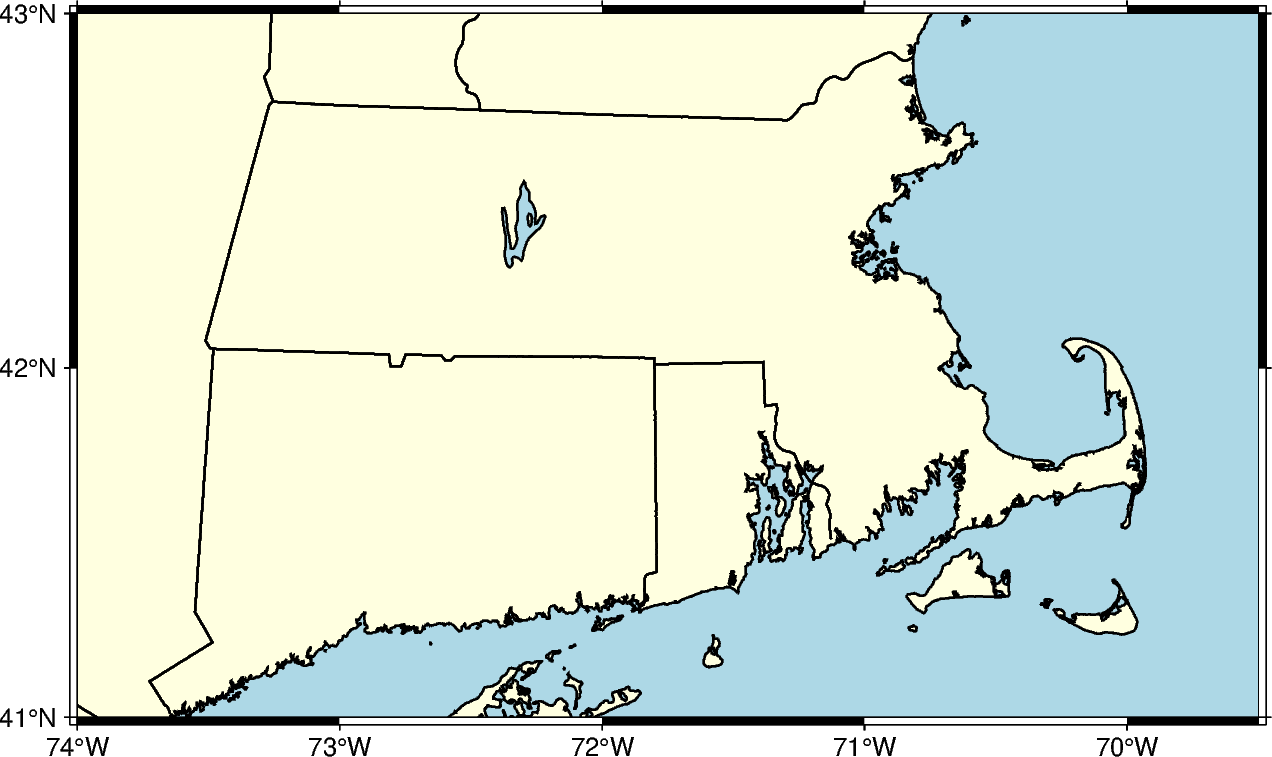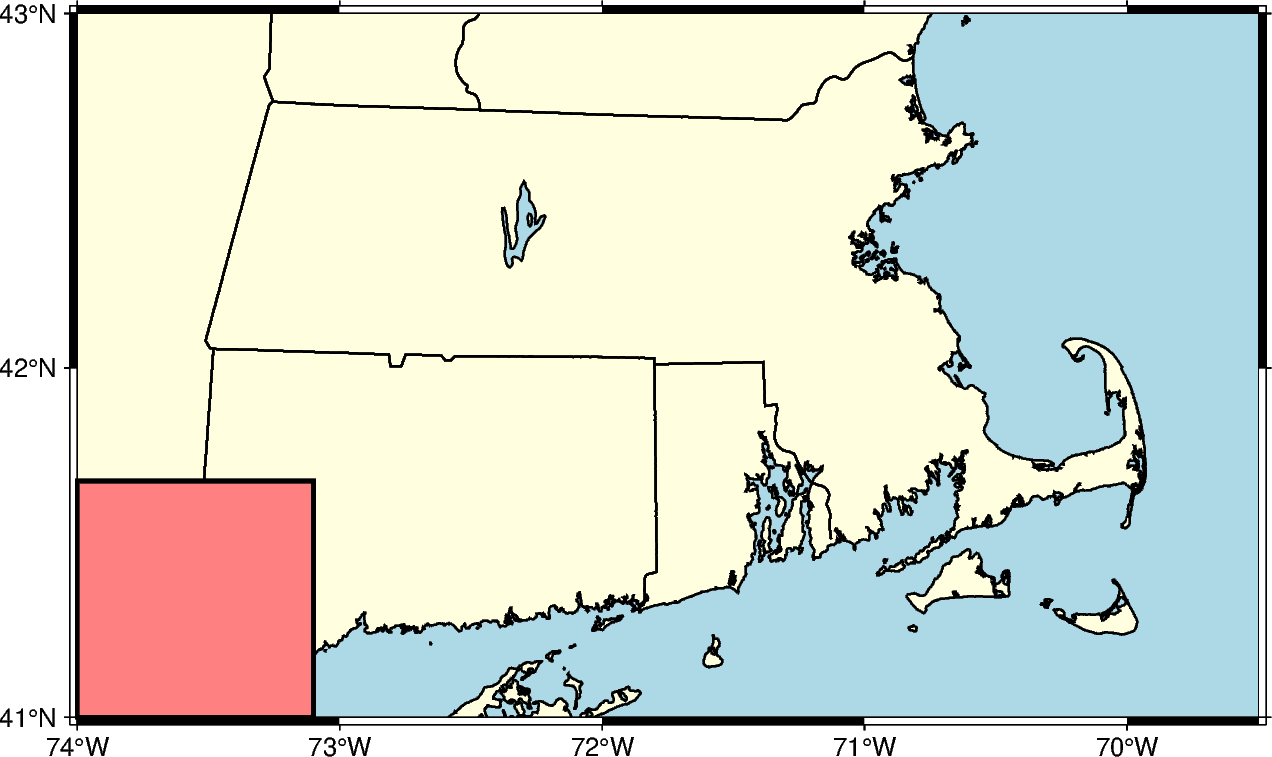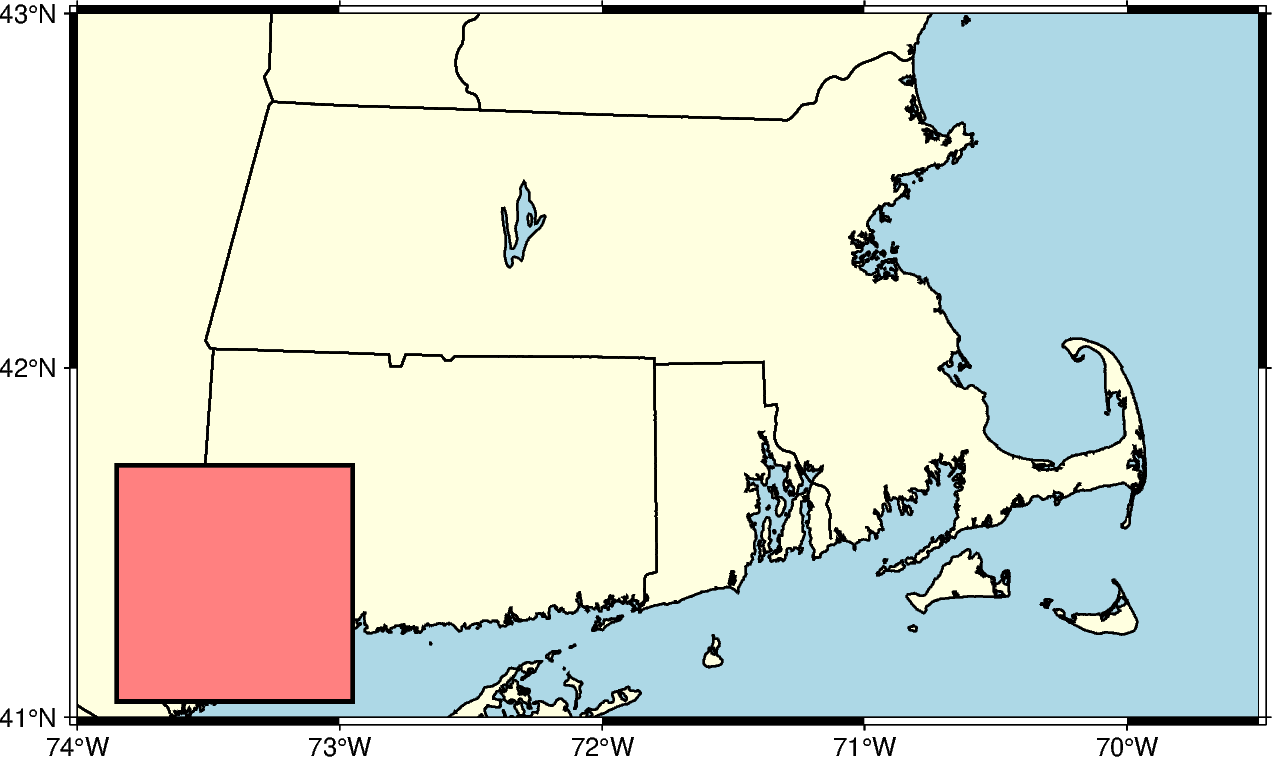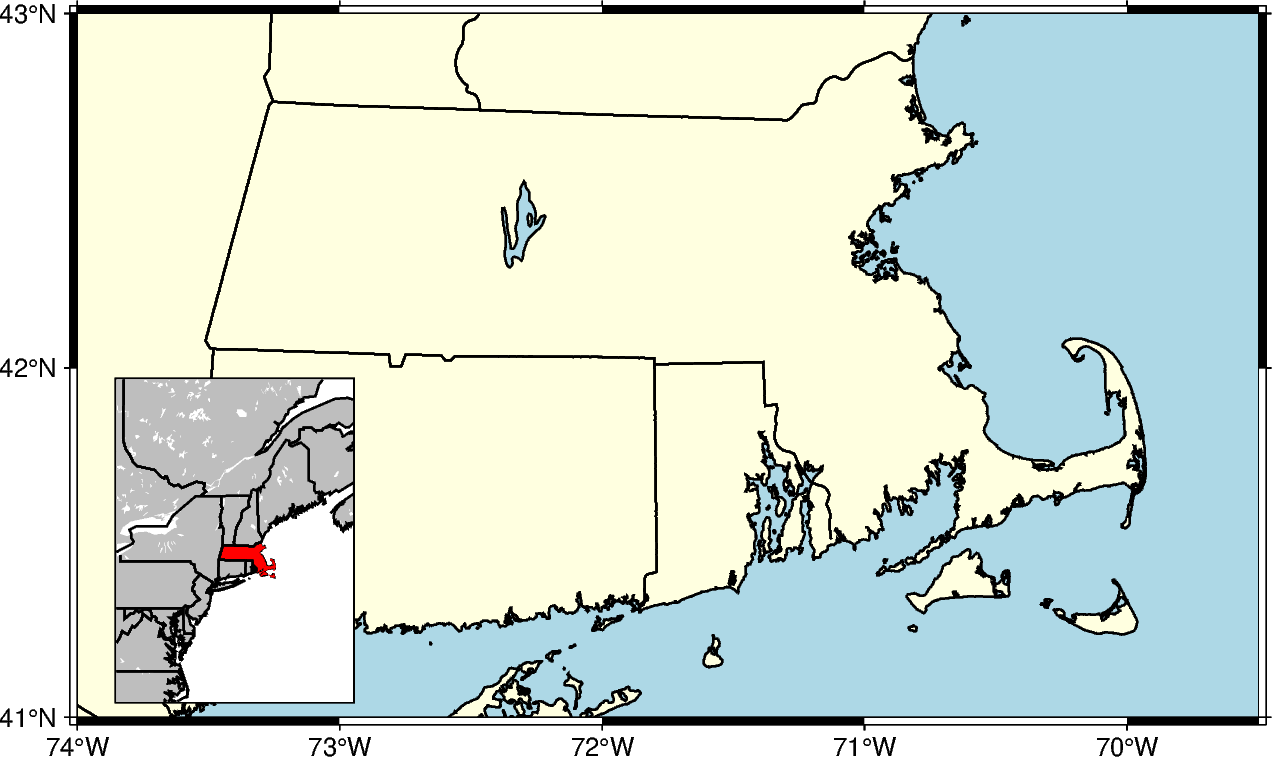# Adding an inset to the figure

To plot an inset figure inside another larger figure, we can use the `pygmt.Figure.inset` method. After a large figure has been created, call `inset` using a `with` statement, and new plot elements will be added to the inset figure instead of the larger figure.

```import pygmt
```

Prior to creating an inset figure, a larger figure must first be plotted. In the example below, `pygmt.Figure.coast` is used to create a map of the US state of Massachusetts.

```fig = pygmt.Figure()
fig.coast(
region=[-74, -69.5, 41, 43],  # Set bounding box of the large figure
borders="2/thin",  # Plot state boundaries with thin lines
shorelines="thin",  # Plot coastline with thin lines
projection="M15c",  # Set Mercator projection and size of 15 centimeter
land="lightyellow",  # Color land areas light yellow
water="lightblue",  # Color water areas light blue
frame="a",  # Set frame with annotation and major tick spacing
)
fig.show()
```The `pygmt.Figure.inset` method uses a context manager, and is called using a `with` statement. The `position` parameter, including the inset width, is required to plot the inset. Using the j argument, the location of the inset is set to one of the 9 anchors (bottom-middle-top and left-center-right). In the example below, `BL` sets the inset to the bottom left. The `box` parameter can set the fill and border of the inset. In the example below, `+pblack` sets the border color to black and `+glightred` sets the fill to light red.

```fig = pygmt.Figure()
fig.coast(
region=[-74, -69.5, 41, 43],
borders="2/thin",
shorelines="thin",
projection="M15c",
land="lightyellow",
water="lightblue",
frame="a",
)
with fig.inset(position="jBL+w3c", box="+pblack+glightred"):
# pass is used to exit the with statement as no plotting methods are
# called
pass
fig.show()
```When using j to set the anchor of the inset, the default location is in contact with the nearby axis or axes. The offset of the inset can be set with +o, followed by the offsets along the x- and y-axis. If only one offset is passed, it is applied to both axes. Each offset can have its own unit. In the example below, the inset is shifted 0.5 centimeters on the x-axis and 0.2 centimeters on the y-axis.

```fig = pygmt.Figure()
fig.coast(
region=[-74, -69.5, 41, 43],
borders="2/thin",
shorelines="thin",
projection="M15c",
land="lightyellow",
water="lightblue",
frame="a",
)
with fig.inset(position="jBL+w3c+o0.5c/0.2c", box="+pblack+glightred"):
pass
fig.show()
```Standard plotting methods can be called from within the `inset` context manager. The example below uses `pygmt.Figure.coast` to plot a zoomed out map that selectively paints the state of Massachusetts to show its location relative to other states.

```fig = pygmt.Figure()
fig.coast(
region=[-74, -69.5, 41, 43],
borders="2/thin",
shorelines="thin",
projection="M15c",
land="lightyellow",
water="lightblue",
frame="a",
)
# This does not include an inset fill as it is covered by the inset figure
# Inset width/height are determined by the ``region`` and ``projection``
# parameters.
with fig.inset(
position="jBL+o0.5c/0.2c",
box="+pblack",
region=[-80, -65, 35, 50],
projection="M3c",
):
# Use a plotting method to create a figure inside the inset.
fig.coast(
land="gray",
borders=[1, 2],
shorelines="1/thin",
water="white",
# Use dcw to selectively highlight an area
dcw="US.MA+gred",
)
fig.show()
```Total running time of the script: ( 0 minutes 2.808 seconds)

Gallery generated by Sphinx-Gallery